红旗，自诞生以来，就熔铸了国人对民族汽车的深厚情谊。
回首中国汽车工业史，没有哪一款车能像红旗轿车那样让人魂牵梦萦。传奇的经历让红旗轿车从诞生起就显得庄严与神圣，它定义了中国高档汽车的内涵，在它的身上演绎了东方神韵与色彩。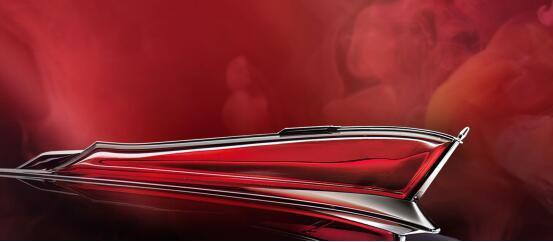其实早在1960年，具有强烈时代精神的红旗CA72就已经蜚声海外，被意大利国际著名造型大师平宁•法里纳誉为“东方艺术与汽车工业技术结合的典范”，并于1960年3月在德国来比锡博览会展出，“红旗”也得以被编入《世界汽车年鉴》，成为世界名车。

时光飞逝，岁月如歌。当2017年8月，徐留平掌舵一汽集团后，上任第一天，他就旗帜鲜明地对内喊话：“红旗一定能在我们这一代人手中实现复兴！”自那之后，扛起红旗振兴的大旗，成为了徐留平心中燃烧的火焰，也是一汽必须实现的目标。
我们看到，在徐留平上任的这4个月里，一汽集团竭尽所能的展现出了大力发展红旗品牌的决心和行动。2017年的最后一天，中国一汽在官微上发布了徐留平的《2018年新年贺词》，新董事长用慷慨激昂的语气，为一汽，为红旗的新一年奠定了拼搏的基调。“2018年，是一汽落实‘四步走’战略、加快推进企业转型升级的基点之年。胸怀梦想，击鼓催征，扛红旗、抓自主、挺创新、强合作，我们将义无反顾。全力以赴！”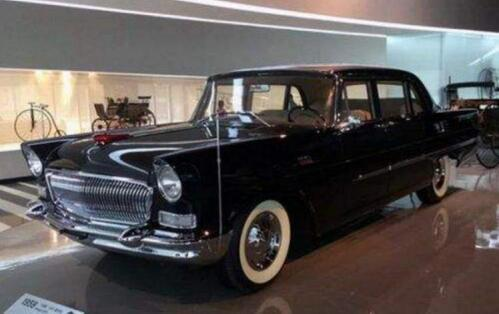2018年1月1日，新年伊始、万象更新！在北京天安门，在嘹亮的升旗号角声中，第一次担任升旗任务的中国人民解放军新仪仗队顺利完成了天安门广场升旗仪式。新仪式、新时代、新变革、新方向，新的强大和自信，展示了新的复兴征程！
就在人们除旧迎新的兴奋中，1月8日，新的红旗梦将在人民大会堂开始！
60年前，红旗轿车带着使命和荣耀驶过人民大会堂。60年后，中国一汽红旗品牌战略发布会又将从人民大会堂传向全世界。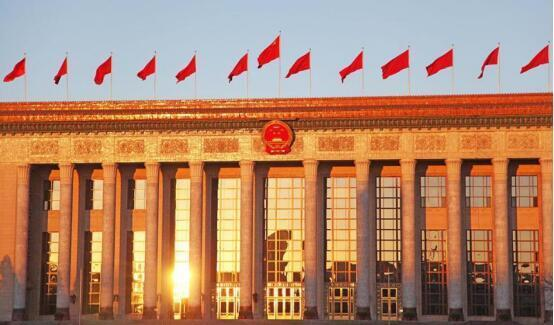这是一场不一样的品牌发布盛会，因为人民大会堂在国人心中具有举足轻重的地位，很多改变国运的决策都是在这里决定。不是所有的汽车品牌能够走进这里，唯有不一样的红旗才有资格从这里发布掷地有声的品牌战略。
这是不一样的红旗汽车，红旗L5将开启限量定制模式，针对量产版产品会提供多达近五十处的产品定制升级，同时更有对应的尊享服务让红旗车主享受中国第一车的荣誉和辉煌。据悉，红旗L5定制版限量100台，每台售价预计超过600万元。L5限量版将完美体现车主高端奢华的身份和地位。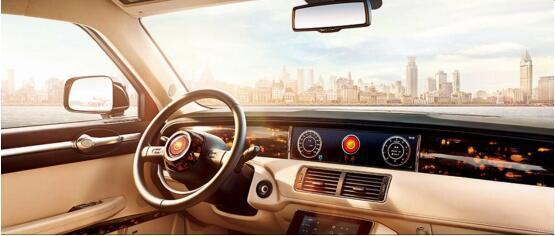更有不一样的嘉宾到场，在1月8日红旗品牌战略发布会举行之时，军党政要、行业精英、名人领袖、媒体和经销商将在人民大会堂汇聚一堂！
那么会有哪些重磅嘉宾呢？其实已经有了剧透。因为在红旗品牌发布会倒计时第10天的时候，一汽发布了这样一张海报“从10佳运动员到新院长，铁榔头一再为梦想定音“，毫无疑问，这应该是郎平，带领中国女排创造传奇的郎平一定会到场！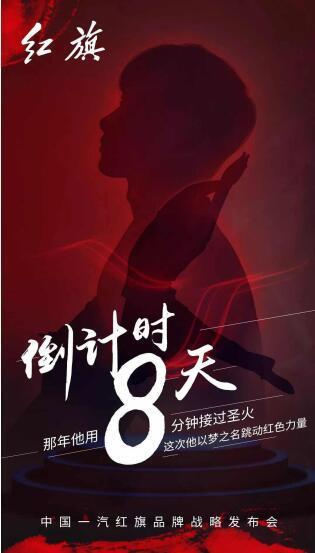倒计时第8天的时候，一汽又发布了一张海报“那年他用8分钟接过圣火，这次他以梦之名跳动红色力量。”这位大咖是谁呢？笔者先不剧透了，大家不妨猜一猜。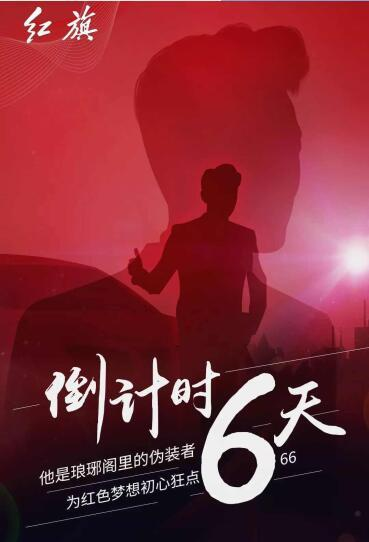倒计时第6天的时候，一汽又发布了“他是琅琊阁的伪装者，为红色梦想初心狂点666。”“琅琊阁？”是一个读书的地方吗？“伪装者？”在讲一名狙击手的故事吗？这位大咖好像很难猜！其实不难，因为这位就是电视剧《琅琊阁》和《伪装者》的主演，而且还是红旗H7的形象代言人！是的，大声说出他的名字吧！他就是——靳东！1月8日，靳东也会到达发布会现场。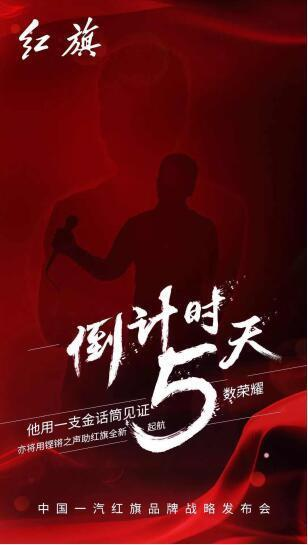而在倒计时第5天的时候，一汽又有了“他用一只金话筒见证无数荣耀，亦将用铿锵之声助红旗全新启航“他又是谁？还是请大家再来猜一猜吧！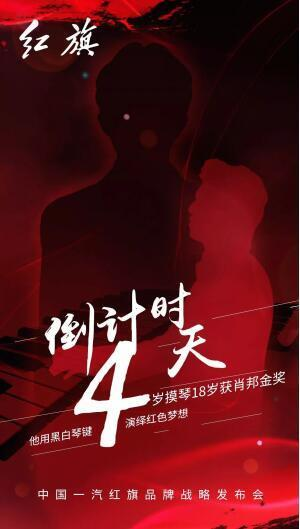就在昨天，又一组倒计时海报放出，“肖邦金奖”、“黑白琴键”有这样的提示后，这个人自然不难猜，但是难猜的是他会与红旗发生什么碰撞呢？

还想知道更多吗？就请继续关注红旗的新海报，更想知道全部消息？那就一定要等到1月8日晚上，关注红旗的发布会直播。届时现场的相关物料、舞美效果都将让人耳目一新，并重新定义中国式豪华的全新风采！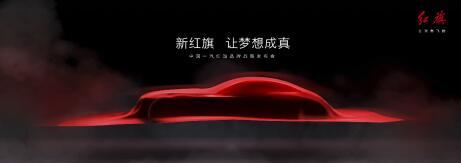我们都知道，当前的中国已经发展成为全球最大的汽车产销国，2017年已经连续6年超越美国成为世界第一汽车大国，但是中国并不是汽车强国，民族汽车工业的发展之路依旧任重道远。
作为中国汽车的一面旗帜，红旗品牌勇于担当，再次挑起了振兴中国汽车工业的大任，从红旗H7再到现在的定制版超豪华汽车L5，红旗的自信前行，是中国汽车梦步向新辉煌的重要开端！

##### 相关资讯

|分享

•已阅！握爪
•我手滑为你点赞
•128个赞！
•不明觉厉
•阅后既醉
•有钱！任性
•照片太美，我不敢看
•干货！杠杠的
•高大上
•膜拜中！
•请接受我的膝盖
•猫哥/猫妹么么哒
•神吐槽
•我只笑笑不说话
•我想静静
•窒息
##### 资讯相关车系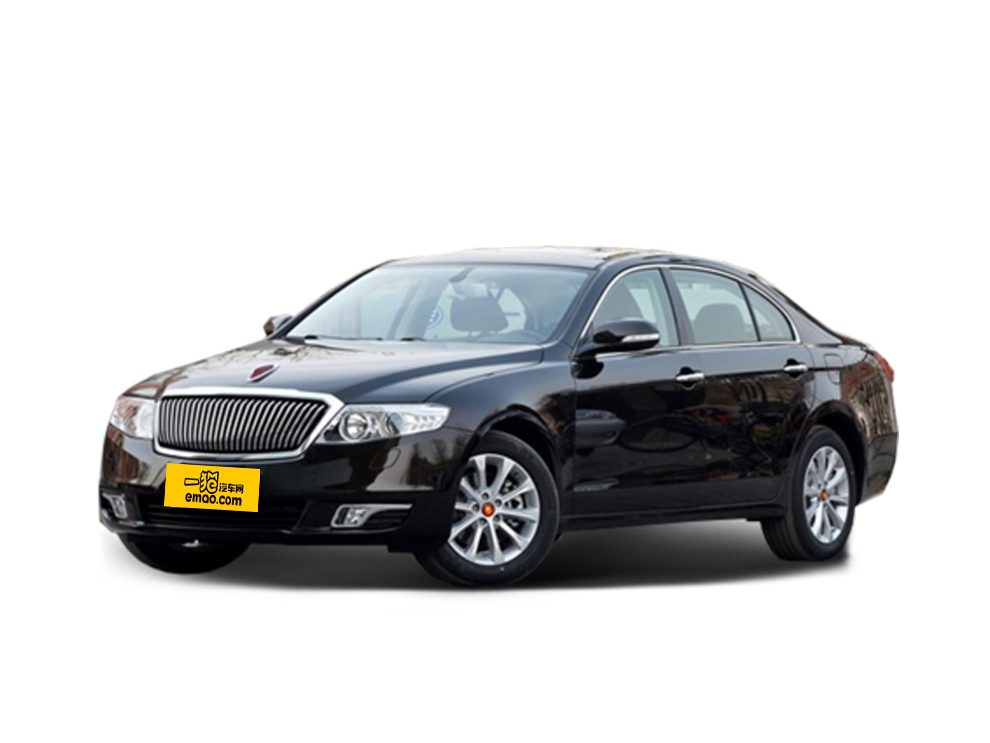##### 热度排行• 阿斯顿·马丁
• 奥迪
• 阿尔法罗密欧
• ALPINA

• 巴博斯
• 宝骏
• 宝马
• 保时捷
• 北汽制造
• 奔驰
• 奔腾
• 本田
• 比亚迪
• 标致
• 别克
• 宾利
• 布加迪
• 北汽威旺
• 北京
• 北汽绅宝
• 北汽幻速
• 北汽新能源
• 宝沃
• 比速汽车
• 北汽道达

• 昌河
• 长安
• 长城
• 长安商用
• 成功汽车
• 长江EV
• 长安轻车型

• 大众
• 道奇
• 东风
• 东风风神
• 东风小康
• 东南
• DS
• 东风风行
• 东风风度
• 东风风光

• 法拉利
• 菲亚特
• 丰田
• 福迪
• 福特
• 福田汽车
• 福汽启腾
• 风诺

• GMC
• 广汽传祺
• 广汽吉奥
• 观致

• 哈飞
• 海格
• 海马
• 华泰
• 黄海
• 恒天
• 红旗
• 哈弗
• 华颂
• 华凯
• 华泰新能源
• 汉腾汽车

• Jeep
• 江淮
• 江铃
• 捷豹
• 金杯
• 九龙
• 吉利汽车
• 金旅
• 金龙
• 江铃集团轻汽
• 江铃集团新能源
• 君马汽车
• 捷途

• 开瑞
• 凯迪拉克
• 科尼赛克
• 克莱斯勒
• KTM
• 卡威
• 凯翼
• 康迪
• 康迪电动汽车集团

• 猎豹汽车
• 兰博基尼
• 劳斯莱斯
• 雷克萨斯
• 雷诺
• 理念
• 力帆
• 莲花汽车
• 林肯
• 铃木
• 陆风
• 路虎
• 路特斯
• 领志
• 领克
• 零跑汽车

• MG
• MINI
• 马自达
• 玛莎拉蒂
• 迈凯伦
• 摩根

• 纳智捷

• 讴歌
• 欧宝
• 欧朗
• 欧拉
• 欧尚汽车

• 帕加尼

• 奇瑞
• 启辰
• 起亚
• 前途
• 庆铃汽车

• 日产
• 荣威
• 瑞麒汽车
• 如虎
• 瑞驰

• 上汽大通
• smart
• 三菱
• 双环
• 双龙
• 斯巴鲁
• 斯柯达
• 萨博
• 思铭
• 赛麟
• SWM斯威汽车

• TESLA
• 泰卡特
• 腾势

• 威麟
• 威兹曼
• 沃尔沃
• 五菱汽车
• 五十铃
• 潍柴英致
• WEY
• 蔚来
• 威马汽车

• 现代
• 雪佛兰
• 雪铁龙
• 西雅特
• 新特汽车
• 小鹏汽车

• 野马汽车
• 一汽
• 依维柯
• 英菲尼迪
• 永源
• 驭胜

• 中华
• 中兴
• 众泰
• 知豆
• 之诺
• 正道汽车
• A
• B
• C
• D
• E
• F
• G
• H
• I
• J
• K
• L
• M
• N
• O
• P
• Q
• R
• S
• T
• U
• V
• W
• X
• Y
• Z

• 阿斯顿·马丁
• 奥迪
• 阿尔法罗密欧
• ALPINA

• 巴博斯
• 宝骏
• 宝马
• 保时捷
• 北汽制造
• 奔驰
• 奔腾
• 本田
• 比亚迪
• 标致
• 别克
• 宾利
• 布加迪
• 北汽威旺
• 北京
• 北汽绅宝
• 北汽幻速
• 北汽新能源
• 宝沃
• 比速汽车
• 北汽道达

• 昌河
• 长安
• 长城
• 长安商用
• 成功汽车
• 长江EV
• 长安轻车型

• 大众
• 道奇
• 东风
• 东风风神
• 东风小康
• 东南
• DS
• 东风风行
• 东风风度
• 东风风光

• 法拉利
• 菲亚特
• 丰田
• 福迪
• 福特
• 福田汽车
• 福汽启腾
• 风诺

• GMC
• 广汽传祺
• 广汽吉奥
• 观致

• 哈飞
• 海格
• 海马
• 华泰
• 黄海
• 恒天
• 红旗
• 哈弗
• 华颂
• 华凯
• 华泰新能源
• 汉腾汽车

• Jeep
• 江淮
• 江铃
• 捷豹
• 金杯
• 九龙
• 吉利汽车
• 金旅
• 金龙
• 江铃集团轻汽
• 江铃集团新能源
• 君马汽车
• 捷途

• 开瑞
• 凯迪拉克
• 科尼赛克
• 克莱斯勒
• KTM
• 卡威
• 凯翼
• 康迪
• 康迪电动汽车集团

• 猎豹汽车
• 兰博基尼
• 劳斯莱斯
• 雷克萨斯
• 雷诺
• 理念
• 力帆
• 莲花汽车
• 林肯
• 铃木
• 陆风
• 路虎
• 路特斯
• 领志
• 领克
• 零跑汽车

• MG
• MINI
• 马自达
• 玛莎拉蒂
• 迈凯伦
• 摩根

• 纳智捷

• 讴歌
• 欧宝
• 欧朗
• 欧拉
• 欧尚汽车

• 帕加尼

• 奇瑞
• 启辰
• 起亚
• 前途
• 庆铃汽车

• 日产
• 荣威
• 瑞麒汽车
• 如虎
• 瑞驰

• 上汽大通
• smart
• 三菱
• 双环
• 双龙
• 斯巴鲁
• 斯柯达
• 萨博
• 思铭
• 赛麟
• SWM斯威汽车

• TESLA
• 泰卡特
• 腾势

• 威麟
• 威兹曼
• 沃尔沃
• 五菱汽车
• 五十铃
• 潍柴英致
• WEY
• 蔚来
• 威马汽车

• 现代
• 雪佛兰
• 雪铁龙
• 西雅特
• 新特汽车
• 小鹏汽车

• 野马汽车
• 一汽
• 依维柯
• 英菲尼迪
• 永源
• 驭胜

• 中华
• 中兴
• 众泰
• 知豆
• 之诺
• 正道汽车
• A
• B
• C
• D
• E
• F
• G
• H
• I
• J
• K
• L
• M
• N
• O
• P
• Q
• R
• S
• T
• U
• V
• W
• X
• Y
• Z

• 阿斯顿·马丁
• 奥迪
• 阿尔法罗密欧
• ALPINA

• 巴博斯
• 宝骏
• 宝马
• 保时捷
• 北汽制造
• 奔驰
• 奔腾
• 本田
• 比亚迪
• 标致
• 别克
• 宾利
• 布加迪
• 北汽威旺
• 北京
• 北汽绅宝
• 北汽幻速
• 北汽新能源
• 宝沃
• 比速汽车
• 北汽道达

• 昌河
• 长安
• 长城
• 长安商用
• 成功汽车
• 长江EV
• 长安轻车型

• 大众
• 道奇
• 东风
• 东风风神
• 东风小康
• 东南
• DS
• 东风风行
• 东风风度
• 东风风光

• 法拉利
• 菲亚特
• 丰田
• 福迪
• 福特
• 福田汽车
• 福汽启腾
• 风诺

• GMC
• 广汽传祺
• 广汽吉奥
• 观致

• 哈飞
• 海格
• 海马
• 华泰
• 黄海
• 恒天
• 红旗
• 哈弗
• 华颂
• 华凯
• 华泰新能源
• 汉腾汽车

• Jeep
• 江淮
• 江铃
• 捷豹
• 金杯
• 九龙
• 吉利汽车
• 金旅
• 金龙
• 江铃集团轻汽
• 江铃集团新能源
• 君马汽车
• 捷途

• 开瑞
• 凯迪拉克
• 科尼赛克
• 克莱斯勒
• KTM
• 卡威
• 凯翼
• 康迪
• 康迪电动汽车集团

• 猎豹汽车
• 兰博基尼
• 劳斯莱斯
• 雷克萨斯
• 雷诺
• 理念
• 力帆
• 莲花汽车
• 林肯
• 铃木
• 陆风
• 路虎
• 路特斯
• 领志
• 领克
• 零跑汽车

• MG
• MINI
• 马自达
• 玛莎拉蒂
• 迈凯伦
• 摩根

• 纳智捷

• 讴歌
• 欧宝
• 欧朗
• 欧拉
• 欧尚汽车

• 帕加尼

• 奇瑞
• 启辰
• 起亚
• 前途
• 庆铃汽车

• 日产
• 荣威
• 瑞麒汽车
• 如虎
• 瑞驰

• 上汽大通
• smart
• 三菱
• 双环
• 双龙
• 斯巴鲁
• 斯柯达
• 萨博
• 思铭
• 赛麟
• SWM斯威汽车

• TESLA
• 泰卡特
• 腾势

• 威麟
• 威兹曼
• 沃尔沃
• 五菱汽车
• 五十铃
• 潍柴英致
• WEY
• 蔚来
• 威马汽车

• 现代
• 雪佛兰
• 雪铁龙
• 西雅特
• 新特汽车
• 小鹏汽车

• 野马汽车
• 一汽
• 依维柯
• 英菲尼迪
• 永源
• 驭胜

• 中华
• 中兴
• 众泰
• 知豆
• 之诺
• 正道汽车
• A
• B
• C
• D
• E
• F
• G
• H
• I
• J
• K
• L
• M
• N
• O
• P
• Q
• R
• S
• T
• U
• V
• W
• X
• Y
• Z